8. REIONIZATION OF THE IGM

When an isolated point source of ionizing radiation turns on, the ionized volume initially grows in size at a rate fixed by the emission of UV photons, and an ionization front separating the H II and H I regions propagates into the neutral gas. Most photons travel freely in the ionized bubble, and are absorbed in a transition layer. The evolution of an expanding H II region is governed by the equation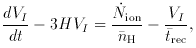(8)

where VI is the proper volume of the ionized zone,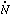ion is the number of ionizing photons emitted by the central source per unit time,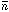H is the mean hydrogen density of the expanding IGM, H is the Hubble constant, and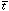rec is the hydrogen mean recombination timescale,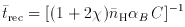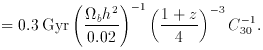(9)

One should point out that the use of a volume-averaged clumping factor, C, in the recombination timescale is only justified when the size of the H II region is large compared to the scale of the clumping, so that the effect of many clumps (filaments) within the ionized volume can be averaged over (see Figure 5). Across the I-front the degree of ionization changes sharply on a distance of the order of the mean free path of an ionizing photon. Whenrec >> t, the growth of the H II region is slowed down by recombinations in the highly inhomogeneous medium, and its evolution can be decoupled from the expansion of the universe. Just like in the static case, the ionized bubble will fill its time-varying Strömgren sphere after a few recombination timescales,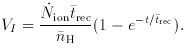(10)

In analogy with the individual H II region case, it can be shown that hydrogen component in a highly inhomogeneous universe is completely reionized when the number of photons emitted above 1 ryd in one recombination time equals the mean number of hydrogen atoms . At any given epoch there is a critical value for the photon emission rate per unit cosmological comoving volume,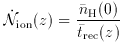(11)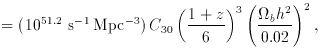independently of the (unknown) previous emission history of the universe: only rates above this value will provide enough UV photons to ionize the IGM by that epoch. One can then compare our estimate ofion to the estimated contribution from QSOs and star-forming galaxies. The uncertainty on this critical rate is difficult to estimate, as it depends on the clumpiness of the IGM (scaled in the expression above to the value inferred at z = 5 from numerical simulations ) and the nucleosynthesis constrained baryon density. The evolution of the critical rate as a function of redshift is plotted in Figure 4 (right). Whileion is comparable to the quasar contribution at z3, there is some indication of a deficit of Lyman continuum photons at z = 5. For bright, massive galaxies to produce enough UV radiation at z = 5, their space density would have to be comparable to the one observed at z3, with most ionizing photons being able to escape freely from the regions of star formation into the IGM. This scenario may be in conflict with direct observations of local starbursts below the Lyman limit showing that at most a few percent of the stellar ionizing radiation produced by these luminous sources actually escapes into the IGM . (4)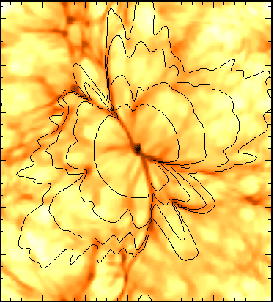Figure 5. Propagation of an ionization front in a 1283 cosmological density field produced by a mini-quasar with= 5 x 1053 s-1. The box length is 2.4 comoving Mpc. The quasar is turned on at the densest cell, which is found in a virialized halo of total mass 1.3 x 1011 M. The solid contours give the position of the I-front at 0.15, 0.25, 0.38, and 0.57 Myr after the quasar has switched on at z = 7. The underlying greyscale image indicates the initial H I density field. (From .)

It is interesting to convert the derived value ofion into a ``minimum'' SFR per unit (comoving) volume,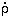* (hereafter we assumebh2 = 0.02 and C = 30):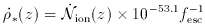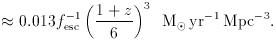(12)

The star formation density given in the equation above is comparable with the value directly ``observed'' (i.e., uncorrected for dust reddening) at z3 . The conversion factor assumes a Salpeter IMF with solar metallicity, and has been computed using a population synthesis code . It can be understood by noting that, for each 1 Mof stars formed, 8% goes into massive stars with M > 20 Mthat dominate the Lyman continuum luminosity of a stellar population. At the end of the C-burning phase, roughly half of the initial mass is converted into helium and carbon, with a mass fraction released as radiation of 0.007. About 25% of the energy radiated away goes into ionizing photons of mean energy 20 eV. For each 1 Mof stars formed every year, we then expect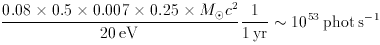(13)

4 Note that, at z = 3, Lyman-break galaxies would radiate more ionizing photons than QSOs for fesc30%. Back.